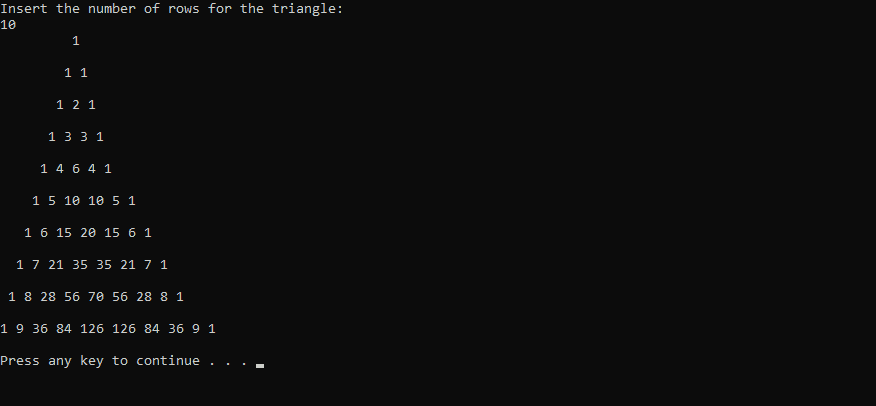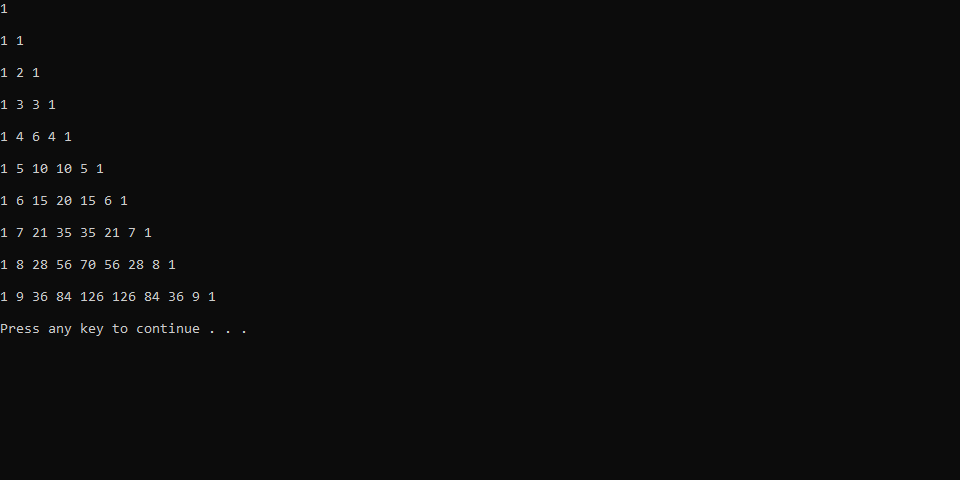# How to print the Pascal's triangle in Swift

In mathematics, Pascal's triangle is a triangular arrangement of numbers that gives the coefficients in the expansion of any binomial expression, such as `(x + y)n`. It is named for the 17th-century French mathematician Blaise Pascal. As an easier explanation for those who are not familiar with binomial expression, the pascal's triangle is a never-ending equilateral triangle of numbers that follow a rule of adding the two numbers above to get the number below.

In this article, we'll show you how to generate this famous triangle in the console with the Swift programming language.

## Printing directly in the console

Graphically, the way to build the pascals triangle is pretty easy, as mentioned, to get the number below you need to add the 2 numbers above and so on:The following code will generate the pascal's triangle:

``````// Function that generates the pascals triangle with a specific number of rows
func generatePascalTriangle(numRows: Int){
var results = [[Int]]()

if (numRows == 0) {
return
}

for i in 0..<numRows {
var currentResults = [Int]()

// Print spaces
for _ in 0..<(numRows - i - 1) {
print(" ", terminator:"")
}

// Print values
for j in 0...i {
if (i > 1 && j > 0 && j < i) {
let value = results[i-1][j] + results[i-1][j-1]
currentResults.append(value)

print("\(value) ", terminator: "")
} else {
currentResults.append(1)
print("\(1) ", terminator: "")
}
}

results.append(currentResults)
print("\n")
}
}

// Prompt the user for the number of rows through the console e.g "10"
print("Insert the number of rows for the triangle:")

// Print the pascals triangle in the console with the given number of rows
generatePascalTriangle(numRows: numberOfRows!)
// Or force the value directly:
// generatePascalTriangle(numRows: 10)``````

The execution of the previous code will generate the following output in the console:## Retrieving triangle data in array

If instead of printing simply the triangle in the console, you may retrieve the data generated by the logic and render it of another way later:

``````// Function that generates the pascals triangle with a specific number of rows
func generate(numRows: Int) -> [[Int]] {
var results = [[Int]]()

if (numRows == 0) {
return results
}

for i in 0..<numRows {
var currentResults = [Int]()

for j in 0...i {
if (i > 1 && j > 0 && j < i) {
let value = results[i-1][j] + results[i-1][j-1]
currentResults.append(value)
} else {
currentResults.append(1)
}
}

results.append(currentResults)
}

return results
}

let triangleContent = generate(numRows: 10)

print(triangleContent)``````

The previous code will generate the following ouput:Remember that you can iterate over the result of the function, for example:

``````let triangleContent = generate(numRows: 10)

for i in 0..<triangleContent.count {
let row = triangleContent[i]

for i in 0..<row.count {
let value = row[i]
print("\(value) ", terminator:"")
}

print("\n")
}``````

The previous snippet will generate the following output:Happy coding !